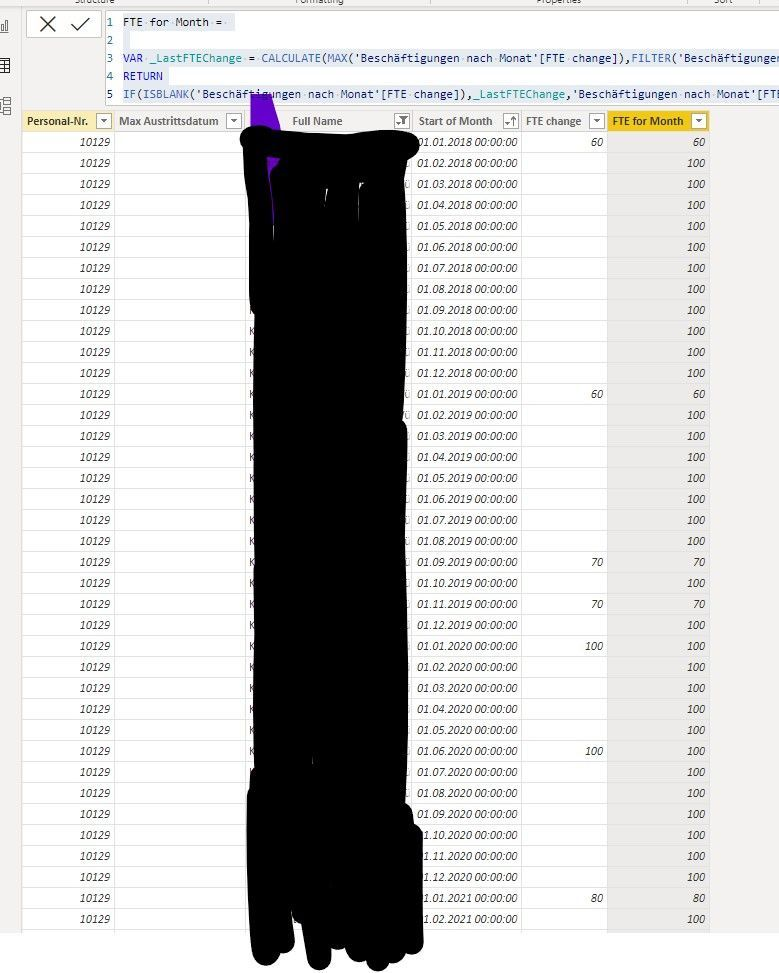cancel
Showing results for
Did you mean:Frequent Visitor

## Populate most recent value until changed

Hi community

I have a calculated table that summarizes employees, months, and their FTE % change. Now I want to create a calculated column that populates the FTE % throughout all months until a new change is detected.

'Start of Month' is the date I am using to create a row for each month

'FTE Change' is showing when an FTE change was made for the employee

'FTE for the month' should now show the latest (earlier?) FTE % until a new value is provided in FTE change. so in the below example: starting with 60, until 1.0.2019 it changes to 70...Tried the following formula but it fills the blanks only with 100 instead of the previous FTE Change, what am I doing wrong?

``````FTE for Month =

VAR _LastFTEChange = CALCULATE(MAX('Beschäftigungen nach Monat'[FTE change]),FILTER('Beschäftigungen nach Monat','Beschäftigungen nach Monat'[Start of Month]<= EARLIER('Beschäftigungen nach Monat'[Start of Month])),'Beschäftigungen nach Monat'[FTE change]>0,ALL('Beschäftigungen nach Monat'))
RETURN
IF(ISBLANK('Beschäftigungen nach Monat'[FTE change]),_LastFTEChange,'Beschäftigungen nach Monat'[FTE change])``````

1 ACCEPTED SOLUTIONSuper User

@CountingPeople Try:

``````Column =
VAR __Value = 'Beschäftigungen nach Monat'[FTE change]
VAR __Date = 'Beschäftigungen nach Monat'[Start of Month]
VAR __PreviousDate = MAXX(FILTER('Beschäftigungen nach Monat', [FTE change] <> BLANK() && [Start of Month] < __Date),[Start of Month])
VAR __PreviousValue = MAXX(FILTER('Beschäftigungen nach Monat',[Start of Month] = __PreviousDate,[FTE change])
VAR __Return = IF('Beschäftigungen nach Monat'[FTE change] = BLANK(),__PreviousValue,__Value)
RETURN
__Return``````

See my article on Mean Time Between Failure (MTBF) which uses EARLIER: http://community.powerbi.com/t5/Community-Blog/Mean-Time-Between-Failure-MTBF-and-Power-BI/ba-p/3395....
The basic pattern is:
Column =
VAR __Current = [Value]
VAR __PreviousDate = MAXX(FILTER('Table','Table'[Date] < EARLIER('Table'[Date])),[Date])

VAR __Previous = MAXX(FILTER('Table',[Date]=__PreviousDate),[Value])
RETURN
__Current - __Previous

Become an expert!: Enterprise DNA
External Tools: MSHGQM
Latest book!:
Mastering Power BI 2nd EditionDAX is easy, CALCULATE makes DAX hard...
3 REPLIES 3Super User

@CountingPeople Try:

``````Column =
VAR __Value = 'Beschäftigungen nach Monat'[FTE change]
VAR __Date = 'Beschäftigungen nach Monat'[Start of Month]
VAR __PreviousDate = MAXX(FILTER('Beschäftigungen nach Monat', [FTE change] <> BLANK() && [Start of Month] < __Date),[Start of Month])
VAR __PreviousValue = MAXX(FILTER('Beschäftigungen nach Monat',[Start of Month] = __PreviousDate,[FTE change])
VAR __Return = IF('Beschäftigungen nach Monat'[FTE change] = BLANK(),__PreviousValue,__Value)
RETURN
__Return``````

See my article on Mean Time Between Failure (MTBF) which uses EARLIER: http://community.powerbi.com/t5/Community-Blog/Mean-Time-Between-Failure-MTBF-and-Power-BI/ba-p/3395....
The basic pattern is:
Column =
VAR __Current = [Value]
VAR __PreviousDate = MAXX(FILTER('Table','Table'[Date] < EARLIER('Table'[Date])),[Date])

VAR __Previous = MAXX(FILTER('Table',[Date]=__PreviousDate),[Value])
RETURN
__Current - __Previous

Become an expert!: Enterprise DNA
External Tools: MSHGQM
Latest book!:
Mastering Power BI 2nd EditionDAX is easy, CALCULATE makes DAX hard...Frequent Visitor

Hi @Greg_Deckler Thanks so much for the help, I think I got the concept!

The calculation still provides some funny results. After some investigations I found that the _previousDate does not provide consistent results:it works for the first few rows, but then somehow fetches the wrong date. It should be 01.01.2018 until the next change.
Does sorting the table have an impact on the way the calculation works?

Thanks again for the help!Frequent Visitor

@Greg_Deckler or anyone, can anyone help and tell me what's going wrong? help is much appreciated!